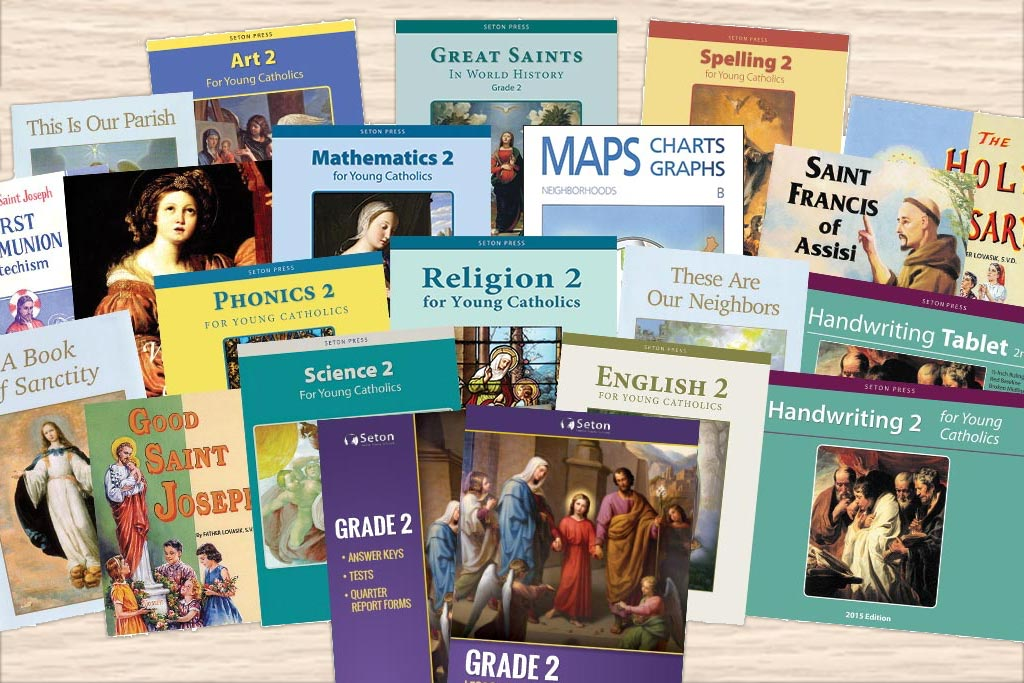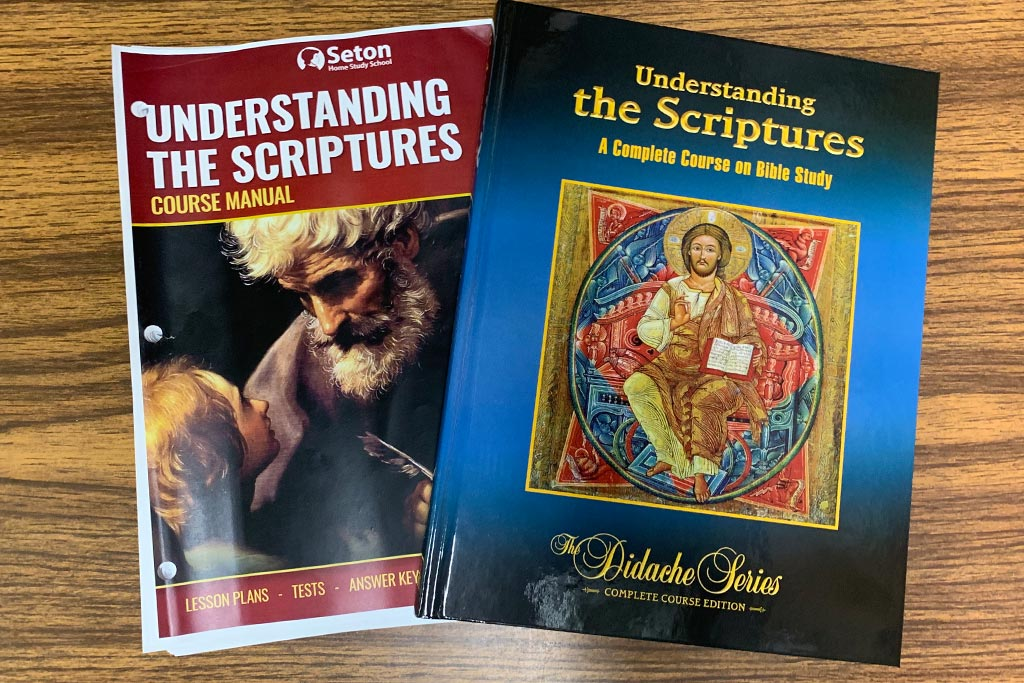Select PageCatholic Homeschool Curriculum

# Seton’s Mathematics Program

Seton offers more options and flexibility in Math than in any other subject. There is a Seton Press Math for Young Catholics workbook series which runs from K to 5, a Saxon series which runs from K to 3, and then another Saxon series (for which there are optional video tutorials) which runs from 5 up to high school, though there are two options in 8th. There is an MCP workbook series which runs from K to 6. Math is also the one subject in elementary in which Seton allows for the use of outside programs that can be incorporated into Seton’s records.

Our usual recommendation and default course listing is Math for Young Catholics for K-5, then Saxon for 6-8 (with the Saxon Algebra 1/2 course instead of the Saxon 8/7 unless the student needs additional review).

## Math Pre-K

In Counting with Numbers, your child will learn to identify, write, and understand the meanings of numbers 1-10. The book introduces concepts such as more, less, empty, full, first, and last.

## Math K

In Mathematics K for Young Catholics, students recognize and distinguish objects, positions, shapes, and patterns, as well as associating numbers and numerals with different sets of items.

Other skills introduced are counting from one to ten, identifying numerals from 0 to 100, and working with lengths and measuring objects. Kindergartners also learn beginning skills in telling time and working with money.

In Mathematics 1 for Young Catholics, students add and subtract using one- and two-digit numbers, recognize and write numerals 0 – 100, and exhibit beginning skills in reasoning and logical thinking, place value, money, time, and are introduced to beginning concepts in geometry, measurement, fractions, and graphing.

## Math 2

Students develop their skills in arithmetic, reasoning, logical thinking, and problem solving in the Mathematics 2 for Young Catholics workbook.

Topics include four-digit place value, adding and subtracting four-digit numbers, geometry, fractions, measurement, and multiplication (through 5 X 5). Students are also introduced to division.

## Math 3

Third graders review addition and subtraction, and continue exploring multiplication and division, including facts through nine.

Other topics covered in Mathematics 3 for Young Catholics include geometry, graphing, fractions, decimals, and solving word problems.

## Math 4

Students improve the accuracy and speed of their problem solving as they practice addition, subtraction, multiplication, and division in Mathematics 4 for Young Catholics.

They also study decimals, adding fractions, graphing, and probability.

## Math 5

In Mathematics 5 for Young Catholics, children attain proficiency in operations with whole numbers, fractions, decimals, and percentages. They receive instruction in basic geometry and measurement, as well as basic concepts related to number theory and order of operations.

## Math 6

Teaching Textbooks Math 6 is an interactive online math course designed specifically for independent learners. The course contains a digital textbook, teacher lectures, and audiovisual step-by-step explanations of math concepts.

Though Seton recommends using the interactive online version, students can also successfully complete the course using the printed textbook alone, which can be purchased instead of, or in addition to, the online license.

In Teaching Textbooks Math 6, students study the following concepts: Whole Numbers, Adding and Subtracting Larger Whole Numbers, Simple Multiplication and Division, Long Multiplication and Long Division, Whole Numbers in the Real World, Fraction Basics, More on Fractions, Working with Factors, Adding and Subtracting Fractions, Multiplying and Dividing Fractions, Fractions in the Real World, Decimal Basics, More on Decimals, Decimals in the Real World, Percents, Geometry, Units of Measure, Graphing Concepts, and more.

##### Alternate: Saxon 65 Home Study Kit

In Saxon Math 65 Home Study Kit, students develop their competency in the four basic operations using fractions and decimals. They exhibit skills in basic geometry and graphing and greater accuracy and understanding in problem-solving.

Students learn prime and composite numbers, ratios, square roots, measurement and unit conversion, the properties of operations, and how to determine perimeter, area, and volume.

The materials for this course incur an additional fee.

## Math 7

Teaching Textbooks Math 7 is an interactive online math course designed specifically for independent learners. The course contains a digital textbook, teacher lectures, and audiovisual step-by-step explanations of math concepts.

Though Seton recommends using the interactive online version, students can also successfully complete the course using the printed textbook alone, which can be purchased instead of, or in addition to, the online license.

In Teaching Textbooks Math 7, students study the following concepts: Adding & Subtracting Whole Numbers, Multiplying & Dividing Whole Numbers, Whole Numbers in the Real World, Fractions, Reducing Fractions, Adding & Subtracting Fractions, Multiplying and Dividing Fractions, Fractions in the Real World, Decimals, Decimals in the Real World, Percents, Percents in the Real World, Geometry Basics, Geometric Measurement, Statistics & Probability, Graphing Concepts, Equations & Inequalities, and more.

##### Alternate: Saxon Math 76(Textbook temporarily unavailable: Only enroll in this course if you already have the required textbook.)

Using Saxon Math 76, students improve their skills in working with fractions, decimals, and percents. They show proficiency in working with time, money, and area; exhibit skill in basic geometry and graphing; and improve their accuracy and understanding in problem-solving.

Students simplify expressions containing parentheses, perform operations with signed numbers, solve word problems with powers and roots, and learn about statistics and probability.

## Pre-Algebra Online

Teaching Textbooks Pre-Algebra is an interactive online math course designed specifically for independent learners. The course contains a digital textbook, teacher lectures, and audiovisual step-by-step explanations of math concepts.

In Teaching Textbooks Pre-Algebra, students study the following concepts: Arithmetic Basics, Rational Numbers, Decimals, Percents, Measuring Length, Measuring Area and Volume, Simple Algebraic Equations, Integers, Longer Algebraic Equations, Combining Like Terms, Rational Expressions, Powers, Polynomials, and Radicals, Geometry, Relations, Functions, and Graphing, Statistics, Probability, Inequalities, and more.

##### Alternate: Saxon Algebra 1/2In Saxon Math Algebra ½, students demonstrate solid arithmetic skills in such areas as factoring, absolute value, signed numbers, order of operations, exponents, ratios, and proportions.

Students learn problem-solving skills related to elementary geometric concepts, basic algebraic concepts, unit multiples, business math, statistics and probability, and develop a basic grasp of rectangular coordinates.#### Full Enrollment

A full enrollment includes all the books you need for a full grade level, along with all of Seton's homeschool services.#### Single Course Enrollment

A single course enrollment includes the books for one course, along with all of Seton's homeschool services.#### Book Sales

Looking for just a few books? Order from Seton Educational Media to buy individual books and supplemental materials.

Your enrollment provides you with much more than just a box of books. It provides an accredited education, a strong support system, and a community of staff and homeschoolers who are in your corner. It provides access to academic and support counseling, grading and record keeping, and a solid Catholic curriculum that promotes strong Catholic values in each and every subject.

#### CATHOLIC MATERIALS

All of our books and materials are thoroughly Catholic, from beginning to end.

#### LESSON PLANS

Your enrollment includes daily lesson plans that are detailed but entirely flexible.

#### ONLINE RESOURCES

Enrolled families can call or email our academic counselors for homeschooling help.